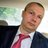## uniform on the sphere [or not]While looking at X validated questions, I came upon this comment that simulating a uniform distribution on a d-dimensional unit sphere does not proceed from generating angles at random on (0,2π) and computing spherical coordinates… Which I must confess would have been my initial suggestion! This is obvious, nonetheless, when computing the Jacobian of the spherical coordinate transform, which involves powers of the sines of the angles, in a decreasing sequence from d-1 to zero. This means that the angles should be simulated according to their respective sine-power densities. However, except for the d=3 case, where simulating from the density sin(φ) is straightforward by inverse cdf, i.e. φ=acos(1-2u), the cdfs for the higher powers are combinations of sines and cosines, and as such are not easily inverted. Take for instance the eighth power:

F⁸(φ)=(840 φ – 672 sin(2 φ) + 168 sin(4 φ) – 32 sin(6 φ) + 3 sin(8 φ))/3072

While the densities are bounded by sin(φ), up to a constant, and hence an accept-reject can be easily derived, the efficiency decreases with the dimension according to the respective ratio of the Wallis’ integrals, unsurprisingly. A quick check for d=4 shows that the Normal simulation+projection-by-division-by-its-norm is faster.Puzzling a bit further about this while running, I wondered at the simultaneous simulations from sin(φ), sin(φ)², sin(φ)³, &tc., but cannot see a faster way to recycle simulations from sin(φ). Points (φ,u) located in-between two adjacent power curves are acceptable simulations from the corresponding upper curve but they need be augmented by points (φ,u) under the lower curve to constitute a representative sample. In the end, this amounts to multiplying simulations from the highest power density as many times as there are powers. No gain in sight… Sigh!However, a few days later, while enjoying the sunset over Mont Blanc(!), I figured out that there exists a direct and efficient way to simulate from these powers of the sine function. Indeed, when looking at the density of cos(φ), it happens to be the signed root of a Beta(½,(d-1)/2), which avoids the accept-reject step. Presumably this is well-known, but I have not seen this proposal associated with the uniform distribution on the sphere.

### 12 Responses to “uniform on the sphere [or not]”

1.Aliaksandr Yemialyan (@AYemialyanau) Says:

Inside the hypersphere

2.Steve Rowley Says:

I realize your real question is how to generate randoms from a pdf which is powers of sines.

But as for the proximal question of how to generate randoms on a hypersphere, here’s how I did it (unsure of how this will render in comments… the lines in the original were up to 95 char wide):

nextVector <- function(N) { # Uniform on 1st 2^N-ant of sphere
## Method of Muller & Marsaglia for generating uniform randoms on the hypersphere:
##
## Muller, M. E. "A Note on a Method for Generating Points Uniformly on N-Dimensional
## Spheres", Comm ACM 2 (1959), 19-20.
## Marsaglia, G. "Choosing a Point from the Surface of a Sphere", Ann Math Stat 43 (1972),
## 645-646. Marsaglia's email is: George Marsaglia .

dotProduct <- function(x, y) { drop(crossprod(x, y)) } # Dot product of 2 vectors

x 1 as N -> +oo, and you waste a lot of work!
} #

•Steve Rowley Says:

Ok, looks like the code was totally mangled by WordPress. Here’s the gist of it, for an N-dimensional hypersphere:

x <- rnorm(N)
abs(x / sqrt(drop(crossprod(x, x))))

•xi'an Says:

Thank you. I am aware of this proposal and so is the person who asked the initial question on X validated.

3.Mario Cortina Borja Says:

Hello,
You may be interested in these papers:

Marsaglia G (1972) Choosing a point from the surface of a sphere.
The Annals of Mathematical Statistics, 43, 645-646

http://chalkdustmagazine.com/blog/sylvesters-convex-hull-problem/

Using Marsaglia’s method, this is a function in R to generate points in the d-dimensional unit ball.

gen.dBall<- function(npts, d)
{
### generate npts points within the d-dimensional unit ball
matd<- matrix( rnorm( npts*d), ncol=d)
d0<- apply(matd, 1, function(x){sqrt(sum(x^2))})
invisible( matd)
}

Best wishes,

Mario

•xi'an Says:

Dear Mario, thanks for the comment. I was aware of George Marsaglia’s paper and method. As stressed in other replies, I am not claiming any novelty in my post or X validated answer, just a resolution to a particular puzzle that I found amusing!

4.You can generate points (x,y,z) uniformly from the surface of a sphere as illustrated below:

n = 500
a = runif(n,0,2*pi)
z = runif(n,-1,1)
r = sqrt(1-z^2)
x = r*cos(a)
y = r*sin(a)

I’ll leave the proof, and possible generalization to higher dimensions, as an exercise for the reader.

•xi'an Says:

Thank you, Radford. The property that one coordinate is marginally uniform is quite helpful to build a recursive algorithm, recursive in the dimension that is.

5.Georges Henry Says:

Pourquoi s’embeter? Si$Z=(Z_1,\ldots,Z_n)$ sont iid N(0,1) alors$Z/\|Z\|$ est uniforme sur la sphere$S_{n-1}$

•xi'an Says:

Je sais, et c’est sûrement plus efficace!, mais bon l’auteur de la question voulait une façon de simuler directement en dimension n-1…

6.coreyyanofsky Says:

The reverse transformation — from polynomials to powers of trig functions — is part of the process of expressing the Beta function in terms of Gamma functions.

•xi'an Says:

Thanks! I deem the simulation method to be less efficient that the x/|x| approach.

This site uses Akismet to reduce spam. Learn how your comment data is processed.